International
Tables for
Crystallography
Volume F
Crystallography of biological macromolecules
Edited by M. G. Rossmann and E. Arnold

International Tables for Crystallography (2006). Vol. F, ch. 13.3, p. 276   | 1 | 2 |

## Section 13.3.3. Patterson-correlation translation function

L. Tonga*

aDepartment of Biological Sciences, Columbia University, New York, NY 10027, USA
Correspondence e-mail: tong@como.bio.columbia.edu

### 13.3.3. Patterson-correlation translation function

| top | pdf |

The most commonly used translation-search indicator is based on the correlation between the observed and the calculated Patterson maps (Crowther & Blow, 1967). Rotation functions are based on the overlap of only a subset of the interatomic vectors in the Patterson map, i.e. only those near the origin of the unit cell, which generally contain the self vectors within each crystallographically unique molecule. The correct orientation and position of a search model in the crystal unit cell should lead to the maximal overlap of both the self and the cross vectors, i.e. maximal overlap between the observed and the calculated Patterson maps throughout the entire unit cell (Tong, 1993),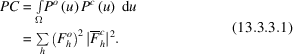The calculated structure factor is a function of the translation vector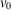[equation (13.3.2.10)]. Combining equations (13.3.2.10) and (13.3.3.1) gives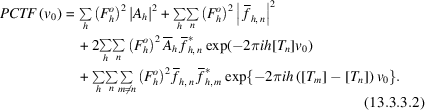The first two terms in equation (13.3.3.2) contribute a constant to the correlation and are generally ignored in this calculation (but see Section 13.3.8). The Patterson-correlation translation function is therefore a Fourier transform, with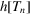(from the third term) and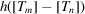(from the fourth term) as the indices. This function can be evaluated quickly by the FFT technique. The termoften leads to the doubling of the original reflection indices. For example,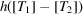will give rise to (2h, 0, 2l) for monoclinic space groups (b-unique setting). Therefore, the Patterson-correlation Fourier transform should normally be sampled with a grid size about 1/6 of the maximum resolution of the reflection data used in the calculation.

A disadvantage of the Patterson-correlation translation function as formulated in equation (13.3.3.2) is that the results from the calculation are on an arbitrary scale. This makes it difficult to compare results from different calculations. The R factor or the correlation coefficient can be calculated for the top peaks in the Patterson-correlation translation function [equation (13.3.3.2)] to place the results on an absolute' scale. Alternatively, the correlation, as defined by equation (13.3.3.1), can be normalized to become a Patterson-correlation coefficient (Harada et al., 1981),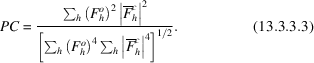This correlation coefficient is equivalent to that defined by equation (13.3.2.3) for reflection intensities (Fujinaga & Read, 1987). The FFT technique can be used to evaluate the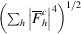term in the denominator of equation (13.3.3.3), which involves reciprocal vectors up to four times the data resolution (Navaza & Vernoslova, 1995). Alternatively, this term can be approximated by an expression which also measures the packing of the search molecules in the crystal (see Section 13.3.5).

The intramolecular vectors can be removed (Crowther & Blow, 1967) from the Patterson maps by subtracting, after appropriate scaling, the structure factors calculated from individual molecules [equation (13.3.2.8)].

The translation functions, as defined above, are based on the structure-factor amplitudes. Normalized structure factors (E's) may provide better results under certain circumstances, since they increase the weight of the high-resolution reflections in the translation function (Harada et al., 1981; Tickle, 1985; Tollin, 1966). Since the Patterson-correlation translation function is also based on reflection intensities, the large term' approach can be used to accelerate the calculation (Tollin & Rossmann, 1966).

### References

Crowther, R. A. & Blow, D. M. (1967). A method of positioning a known molecule in an unknown crystal structure. Acta Cryst. 23, 544–548.Google Scholar
Fujinaga, M. & Read, R. J. (1987). Experiences with a new translation-function program. J. Appl. Cryst. 20, 517–521.Google Scholar
Harada, Y., Lifchitz, A., Berthou, J. & Jolles, P. (1981). A translation function combining packing and diffraction information: an application to lysozyme (high-temperature form). Acta Cryst. A37, 398–406.Google Scholar
Navaza, J. & Vernoslova, E. (1995). On the fast translation functions for molecular replacement. Acta Cryst. A51, 445–449.Google Scholar
Tickle, I. J. (1985). Review of space group general translation functions that make use of known structure information and can be expanded as Fourier series. In Proceedings of the Daresbury study weekend. Molecular replacement, edited by P. A. Machin, pp. 22–26. Warrington: Daresbury Laboratory.Google Scholar
Tollin, P. (1966). On the determination of molecular location. Acta Cryst. 21, 613–614.Google Scholar
Tollin, P. & Rossmann, M. G. (1966). A description of various rotation function programs. Acta Cryst. 21, 872–876.Google Scholar
Tong, L. (1993). Replace, a suite of computer programs for molecular-replacement calculations. J. Appl. Cryst. 26, 748–751.Google Scholar